Oxidation-Reduction Reactions

Oxidation-Reduction Reactions

The Process of Discovery: Oxidation and Reduction

The first step toward a theory of chemical reactions was taken by Georg Ernst Stahl in 1697 when he proposed the phlogiston theory, which was based on the following observations.

• Metals have many properties in common.
• Metals often produce a "calx" when heated. (The term calx is defined as the crumbly residue left after a mineral or metal is roasted.)
• These calxes are not as dense as the metals from which they are produced.
• Some of these calxes form metals when heated with charcoal.
• With only a few exceptions, the calx is found in nature, not the metal.

These observations led Stahl to the following conclusions.

• Phlogiston (from the Greek phlogistos, "to burn") is given off whenever something burns.
• Wood and charcoal are particularly rich in phlogiston because they leave very little ash when they burn. (Candles must be almost pure phlogiston because they leave no ash.)
• Because they are found in nature, calxes must be simpler than metals.
• Metals form a calx by giving off phlogiston.

Metal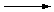calx + phlogiston

Calx + phlogistonmetal

• Because charcoal is rich in phlogiston, heating calxes in the presence of charcoal sometimes produces metals.

This model was remarkably successful. It explained why metals have similar propertiesthey all contained phlogiston. It explained the relationship between metals and their calxesthey were related by the gain or loss of phlogiston. It even explained why a candle goes out when placed in a bell jarthe air eventually becomes saturated with phlogiston.

There was only one problem with the phlogiston theory. As early as 1630, Jean Rey noted that tin gains weight when it forms a calx. (The calx is about 25% heavier than the metal.) From our point of view, this seems to be a fatal flaw: If phlogiston is given off when a metal forms a calx, why does the calx weigh more than the metal? This observation didn't bother proponents of the phlogiston theory. Stahl explained it by suggesting that the weight increased because air entered the metal to fill the vacuum left after the phlogiston escaped.

The phlogiston theory was the basis for research in chemistry for most of the 18th century. It was not until 1772 that Antoine Lavoisier noted that nonmetals gain large amounts of weight when burned in air. (The weight of phosphorus, for example, increases by a factor of about 2.3.) The magnitude of this change led Lavoisier to conclude that phosphorus must combine with something in air when it burns. This conclusion was reinforced by the observation that the volume of air decreases by a factor of 1/5th when phosphorus burns in a limited amount of air.

Lavoisier proposed the name oxygene (literally, "acid-former") for the substance absorbed from air when a compound burns. He chose this name because the products of the combustion of nonmetals such as phosphorus are acids when they dissolve in water.

 P4(s) + 5 O2(g)P4O10(s) P4O10(s) + 6 H2O(l)4 H3PO4(aq)

Lavoisier's oxygen theory of combustion was eventually accepted and chemists began to describe any reaction between an element or compound and oxygen as oxidation. The reaction between magnesium metal and oxygen, for example, involves the oxidation of magnesium.

2 Mg(s) + O2(g)2 MgO(s)

By the turn of the 20th century, it seemed that all oxidation reactions had one thing in commonoxidation always seemed to involve the loss of electrons. Chemists therefore developed a model for these reactions that focused on the transfer of electrons. Magnesium metal, for example, was thought to lose electrons to form Mg2+ ions when it reacted with oxygen. By convention, the element or compound that gained these electrons was said to undergo reduction. In this case, O2 molecules were said to be reduced to form O2- ions.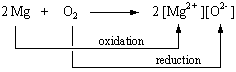A classic demonstration of oxidation-reduction reactions involves placing a piece of copper wire into an aqueous solution of the Ag+ ion. The reaction involves the net transfer of electrons from copper metal to Ag+ ions to produce whiskers of silver metal that grow out from the copper wire and Cu2+ ions.

Cu(s) + 2 Ag+(aq)Cu2+(aq) + 2 Ag(s)

The Cu2+ ions formed in this reaction are responsible for the light-blue color of the solution. Their presence can be confirmed by adding ammonia to this solution to form the deep-blue Cu(NH3)42+ complex ion.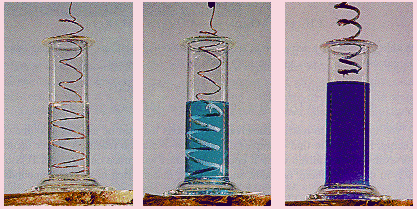Chemists eventually recognized that oxidation-reduction reactions don't always involve the transfer of electrons. There is no change in the number of valence electrons on any of the atoms when CO2 reacts with H2, for example,

CO2(g) + H2(g)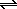CO(g) + H2O(g)

as shown by the following Lewis structures: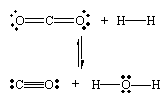Chemists therefore developed the concept of oxidation number to extend the idea of oxidation and reduction to reactions in which electrons are not really gained or lost. The most powerful model of oxidation-reduction reactions is based on the following definitions.

Oxidation involves an increase in the oxidation number of an atom.

Reduction occurs when the oxidation number of an atom decreases.

According to this model, CO2 is reduced when it reacts with hydrogen because the oxidation number of the carbon decreases from +4 to +2. Hydrogen is oxidized in this reaction because its oxidation number increases from 0 to +1.Oxidation-Reduction Reactions

We find examples of oxidation-reduction or redox reactions almost every time we analyze the reactions used as sources of either heat or work. When natural gas burns, for example, an oxidation-reduction reaction occurs that releases more than 800 kJ/mol of energy.

CH4(g) + 2 O2(g)CO2(g) + 2 H2O(g)

Within our bodies, a sequence of oxidation-reduction reactions are used to burn sugars, such as glucose (C6H12O6) and the fatty acids in the fats we eat.

 C6H12O6(aq) + 6 O2(g)6 CO2(g) + 6 H2O(l) CH3(CH2)16CO2H(aq) + 26 O2(g)18 CO2(g) + 18 H2O(l)

We don't have to restrict ourselves to reactions that can be used as a source of energy, however, to find examples of oxidation-reduction reactions. Silver metal, for example, is oxidized when it comes in contact with trace quantities of H2S or SO2 in the atmosphere or foods, such as eggs, that are rich in sulfur compounds.

4 Ag(s) + 2 H2S(g) + O2(g)2 Ag2S(s) + 2 H2O(g)

Fortunately, the film of Ag2S that collects on the metal surface forms a protective coating that slows down further oxidation of the silver metal.

The tarnishing of silver is just one example of a broad class of oxidation-reduction reactions that fall under the general heading of corrosion. Another example is the series of reactions that occur when iron or steel rusts. When heated, iron reacts with oxygen to form a mixture of iron(II) and iron(III) oxides.

 2 Fe(s) + O2(g)2 FeO(s) 2 Fe(s) + 3 O2(g)2 Fe2O3(s)

Molten iron even reacts with water to form an aqueous solution of Fe2+ ions and H2 gas.

Fe(l) + 2 H2O(l)Fe2+(aq) + 2 OH-(aq) + H2(g)

At room temperature, however, all three of these reactions are so slow they can be ignored.

Iron only corrodes at room temperature in the presence of both oxygen and water. In the course of this reaction, the iron is oxidized to give a hydrated form of iron(II) oxide.

2 Fe(s) + O2(aq) + 2 H2O(l)2 FeO H2O(s)

Because this compound has the same empirical formula as Fe(OH)2, it is often mistakenly called iron(II), or ferrous, hydroxide. The FeO H2O formed in this reaction is further oxidized by O2 dissolved in water to give a hydrated form of iron(III), or ferric, oxide.

4 FeO H2O(s) + O2(aq) + 2 H2O(l)2 Fe2O3 3 H2O(s)

To further complicate matters, FeO H2O formed at the metal surface combines with Fe2O3 3 H2O to give a hydrated form of magnetic iron oxide (Fe3O4).

FeO H2O(s) + Fe2O33 H2O(s) Fe3O4 n H2O(s)

Because these reactions only occur in the presence of both water and oxygen, cars tend to rust where water collects. Furthermore, because the simplest way of preventing iron from rusting is to coat the metal so that it doesn't come in contact with water, cars were originally painted for only one reasonto slow down the formation of rust.Assigning Oxidation Numbers

The key to identifying oxidation-reduction reactions is recognizing when a chemical reaction leads to a change in the oxidation number of one or more atoms. It is therefore a good idea to take another look at the rules for assigning oxidation numbers. By definition, the oxidation number of an atom is equal to the charge that would be present on the atom if the compound was composed of ions. If we assume that CH4 contains C4- and H+ ions, for example, the oxidation numbers of the carbon and hydrogen atoms would be -4 and +1.

Note that it doesn't matter whether the compound actually contains ions. The oxidation number is the charge an atom would have if the compound was ionic. The concept of oxidation number is nothing more than a bookkeeping system used to keep track of electrons in chemical reactions. This system is based on a series of rules, summarized in the table below.

 Rules for Assigning Oxidation Numbers The oxidation number of an atom is zero in a neutral substance that contains atoms of only one element. Thus, the atoms in O2, O3, P4, S8, and aluminum metal all have an oxidation number of 0. The oxidation number of monatomic ions is equal to the charge on the ion. The oxidation number of sodium in the Na+ ion is +1, for example, and the oxidation number of chlorine in the Cl- ion is -1. The oxidation number of hydrogen is +1 when it is combined with a nonmetal. Hydrogen is therefore in the +1 oxidation state in CH4, NH3, H2O, and HCl. The oxidation number of hydrogen is -1 when it is combined with a metal. Hydrogen is therefore in the -1 oxidation state in LiH, NaH, CaH2, and LiAlH4. The metals in Group IA form compounds (such as Li3N and Na2S) in which the metal atom is in the +1 oxidation state. The elements in Group IIA form compounds (such as Mg3N2 and CaCO3) in which the metal atom is in the +2 oxidation state. Oxygen usually has an oxidation number of -2. Exceptions include molecules and polyatomic ions that contain O-O bonds, such as O2, O3, H2O2, and the O22- ion. The nonmetals in Group VIIA often form compounds (such as AlF3, HCl, and ZnBr2) in which the nonmetal is in the -1 oxidation state. The sum of the oxidation numbers of the atoms in a molecule is equal to the charge on the molecule. The most electronegative element in a compound has a negative oxidation number.

Any set of rules, no matter how good, will only get you so far. You then have to rely on a combination of common sense and prior knowledge. Questions to keep in mind while assigning oxidation numbers include the following: Are there any recognizable ions hidden in the molecule? Does the oxidation number make sense in terms of the known electron configuration of the atom?

 Practice Problem 1:Determine the oxidation number of each element in the following compounds: (a) BaO2     (b) (NH4)2MoO4      (c)Na3Co(NO2)6      (d) CS2 Click here to check your answer to Practice Problem 1. Click here to see a solution to Practice Problem 1.Recognizing Oxidation-Reduction Reactions

Chemical reactions are often divided into two categories: oxidation-reduction or metathesis reactions. Metathesis reactions include acid-base reactions that involve the transfer of an H+ ion from a Brnsted acid to a Brnsted base.

 CH3CO2H(aq) + OH-(aq)CH3CO2-(aq) + H2O(l) Brnsted acid Brnsted base Brnsted base Brnsted acid

They can also involve the sharing of a pair of electrons by an electron-pair donor (Lewis base) and an electron-pair acceptor (Lewis acid).

 Co3+(aq) + 6 NO2-(aq)Co(NO2)63-(aq) Lewis acid Lewis base

Oxidation-reduction reactions or redox reactions can involve the transfer of one or more electrons.

Cu(s) + 2 Ag+(aq)Cu2+(aq) + 2 Ag(s)

They can also occur by the transfer of oxygen, hydrogen, or halogen atoms.

 CO2(g) + H2(g)CO(g) + H2O(g) SF4(g) + F2(g)SF6(g)

Fortunately, there is an almost foolproof method of distinguishing between metathesis and redox reactions. Reactions in which none of the atoms undergoes a change in oxidation number are metathesis reactions. There is no change in the oxidation number of any atom in either of the metathesis reactions, for example.The word metathesis literally means "interchange" or "transposition," and it is used to describe changes that occur in the order of letters or sounds in a word as a language develops. Metathesis occurred, for example, when the Old English word brid became bird. In chemistry, metathesis is used to describe reactions that interchange atoms or groups of atoms between molecules.

When at least one atom undergoes a change in oxidation state, the reaction is an oxidation-reduction reaction. Each of the reactions in the figure below is therefore an example of an oxidation-reduction reaction.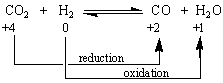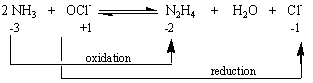Practice Problem 2:Classify each of the following as either a metathesis or an oxidation-reduction reaction.   Note that mercury usually exists in one of three oxidation states: mercury metal, Hg22+ ions, or Hg2+ ions. (a) Hg22+(aq) + 2 OH-(aq)Hg2O(s) + H2O(l) (b) Hg22+(aq)  + Sn2+(aq)2 Hg(l) + Sn4+(aq) (c) Hg22+(aq) + H2S(aq)Hg(l) + HgS(s) + 2 H+(aq) (d) Hg2CrO4(s) + 2 OH-(aq)Hg2O(s) + CrO42-(aq) + H2O(l) Click here to check your answer to Practice Problem 2. Click here to see a solution to Practice Problem 2.